Swift Talk # 291

### This episode is freely available thanks to the support of our subscribers

We prepare a tree diagram view in SwiftUI to experiment with advanced alignment techniques.

00:06 Today, we'll once again draw some tree diagrams. In our last series on this topic, we manually computed a tree's node positions using the Knuth algorithm. That was interesting, but it was also a lot of work. This time, we want to use built-in components like `HStack` and `VStack` in combination with alignment guides to lay out a tree diagram.

01:00 This will be a case study of what we can create using stack views and alignment guides. But before we get to the more complex stuff, we have to prepare a few things, as we'll be building everything up from scratch.

## Getting Started

01:26 The first thing we need is a simple `Tree` structure. We make this struct generic over the type of value it holds. To easily create trees, we add an initializer that skips the `value` label and provides a default empty array for the `children` argument:

``````struct Tree<A> {
init(_ value: A, children: [Tree<A>] = []) {
self.value = value
self.children = children
}

var value: A
var children: [Tree<A>] = []
}
``````

01:56 We construct a sample tree:

``````let sample = Tree("Root", children: [
Tree("First Child"),
Tree("Second"),
])
``````

02:14 The next step is drawing the tree. We write a `Diagram` view, which is generic over the tree's value type. The view takes a view builder that can turn a single value into a node view. For the body view, we run the view builder with — for now — just the tree's root value:

``````struct Diagram<A, Node: View>: View {
var tree: Tree<A>
@ViewBuilder var node: (A) -> Node

var body: some View {
node(tree.value)
}
}
``````

03:11 We add a `Diagram` view with the sample tree to `ContentView`. For the diagram's node view builder, we return a `Text` with the node's string value. We use the `fixedSize` modifier so that the text view renders its string at its ideal width. We also give the text view some padding and a background color:

``````struct ContentView: View {
var body: some View {
Diagram(tree: sample) { value in
Text(value)
.fixedSize()
.background(.tertiary)
}
.frame(maxWidth: .infinity, maxHeight: .infinity)
}
}
``````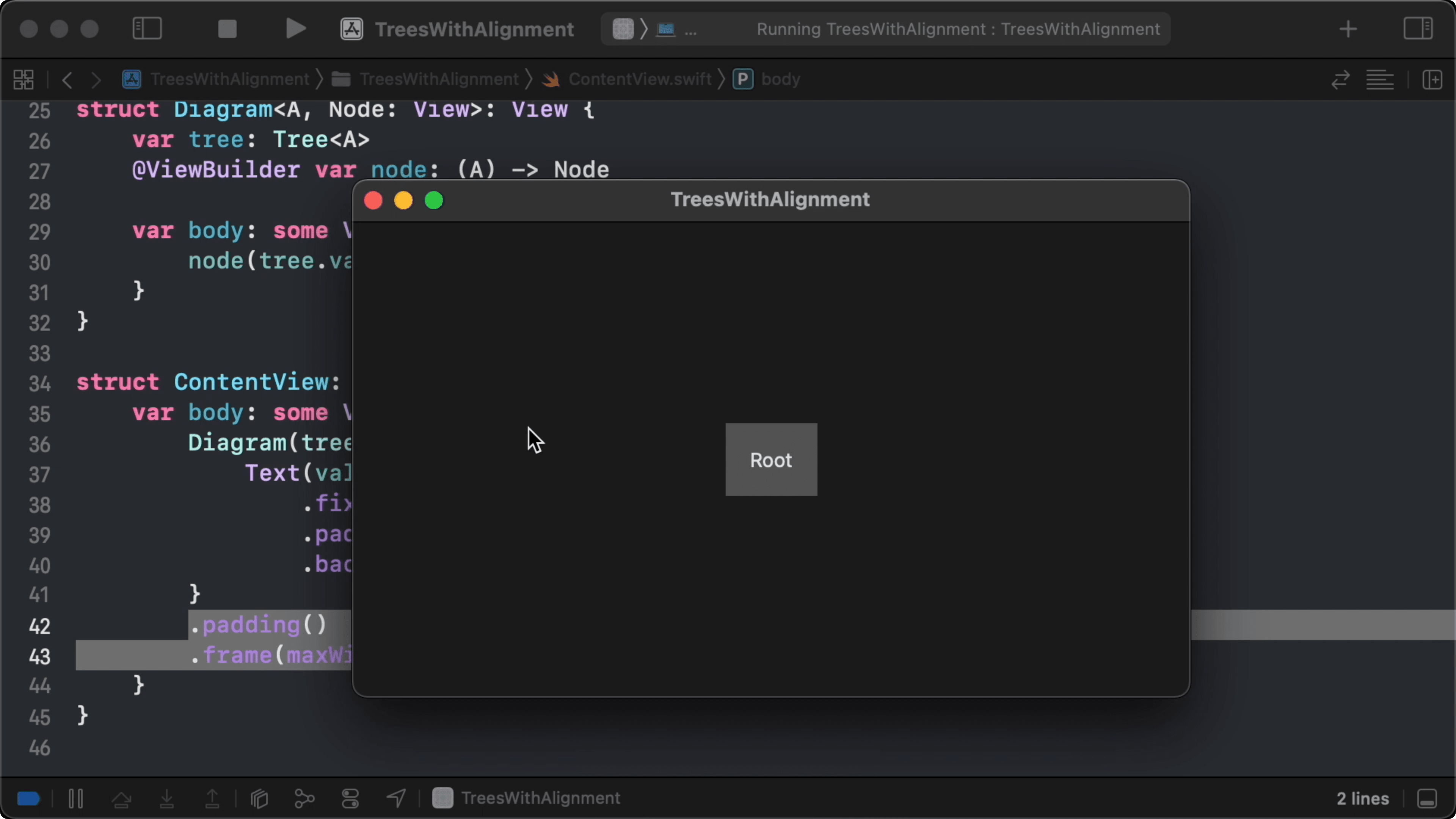## Rendering Child Nodes

03:45 To draw the tree's child nodes below the root node, we wrap the root in a `VStack`, together with an `HStack` containing the children. We render the children recursively by adding a `Diagram` to the `HStack` for each child, passing on the `node` view builder:

``````struct Diagram<A, Node: View>: View {
var tree: Tree<A>
@ViewBuilder var node: (A) -> Node

var body: some View {
VStack {
node(tree.value)
HStack {
ForEach(tree.children) { child in
Diagram(tree: child, node: node)
}
}
}
}
}
``````

04:42 To make `ForEach` happy, we conform `Tree` to `Identifiable`:

``````struct Tree<A>: Identifiable {
init(_ value: A, children: [Tree<A>] = []) {
self.value = value
self.children = children
}

var value: A
var children: [Tree<A>] = []
let id = UUID()
}
``````

04:59 We give the `HStack` and the `VStack` some uniform spacing:

``````struct Diagram<A, Node: View>: View {
var tree: Tree<A>
@ViewBuilder var node: (A) -> Node

var body: some View {
VStack(spacing: 20) {
node(tree.value)
HStack(spacing: 20) {
ForEach(tree.children) { child in
Diagram(tree: child, node: node)
}
}
}
}
}
``````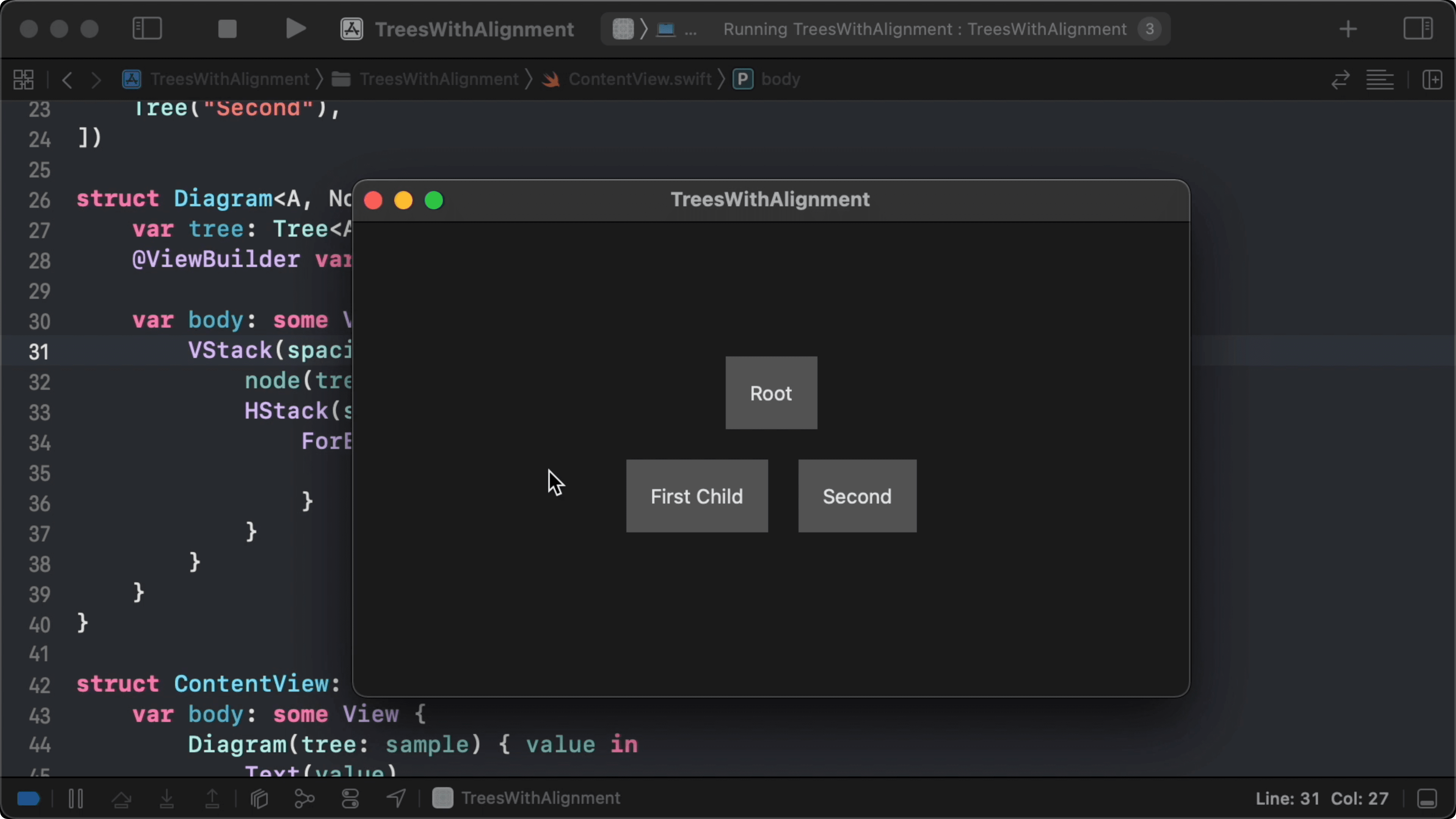## Drawing Lines

05:25 The root node is centered above the combined frame of its children. When we draw lines from the root to the children, we'll see that these lines aren't symmetrical, which looks a little odd.

06:07 First, we write a `Line` shape:

``````struct Line: Shape {
var from: CGPoint
var to: CGPoint

func path(in rect: CGRect) -> Path {
Path { p in
p.move(to: from)
}
}
}
``````

06:50 To draw lines between the root node and each of the child nodes, we need to know the frames of all nodes in a common coordinate space. We can create this common coordinate space by calling `coordinateSpace` on the outer stack view:

``````struct Diagram<A, Node: View>: View {
var tree: Tree<A>
@ViewBuilder var node: (A) -> Node

let coordinateSpace = "diagram"

var body: some View {
VStack(spacing: 20) {
node(tree.value)
HStack(spacing: 20) {
ForEach(tree.children) { child in
Diagram(tree: child, node: node)
}
}
}
.coordinateSpace(name: coordinateSpace)
}
}
``````

08:03 Next, we'll write a helper that measures a view's frame and propagates it up the view tree along with the identifier of the view's node. We pass in the node identifiers so that we can later find a specific node's frame in a collection of measured frames:

``````struct Diagram<A, Node: View>: View {
var tree: Tree<A>
@ViewBuilder var node: (A) -> Node

let coordinateSpace = "diagram"

var body: some View {
VStack(spacing: 20) {
node(tree.value)
.measureFrame(in: .named(coordinateSpace), id: tree.id)
HStack(spacing: 20) {
ForEach(tree.children) { child in
Diagram(tree: child, node: node)
.measureFrame(in: .named(coordinateSpace), id: child.id)
}
}
}
.coordinateSpace(name: coordinateSpace)
}
}
``````

08:39 We write a preference key to store a dictionary of `UUID` and `CGRect`. Its default value is an empty dictionary, and its combine function merges two dictionaries:

``````struct FrameKey: PreferenceKey {
static var defaultValue: [UUID: CGRect] { [:] }
static func reduce(value: inout [UUID : CGRect], nextValue: () -> [UUID : CGRect]) {
value.merge(nextValue(), uniquingKeysWith: { \$1 })
}
}
``````

09:19 The `measureFrame` helper method takes a coordinate space and a `UUID`. It then uses a geometry reader to measure the view's frame, and it stores this frame in a preference:

``````extension View {
func measureFrame(in coordinateSpace: CoordinateSpace, id: UUID) -> some View {
Color.clear.preference(key: FrameKey.self, value: [id: proxy.frame(in: coordinateSpace)])
})
}
}
``````

10:35 All these preferences are propagated up the view tree and combined into a single dictionary. We usually call `onPreferenceChanged` to read the dictionary and store it in a state property, but there's another API we can use. It takes a preference value and produces a background view from it, which fits our use case perfectly:

``````.backgroundPreferenceValue(FrameKey.self) { frames in
// build some `View` using `frames`
}
``````

11:44 The dictionary contains the frames of the root node and the child nodes. We want to draw a line from the root node to each of the children, so we first look up the root node's frame. Then, we filter the root node out of the frames dictionary to get an array of the child frames.

13:00 We have to help the compiler out by explicitly stating which overload of `filter` we want to use — i.e. the one that returns an array of key-value tuples instead of a new dictionary:

``````struct Diagram<A, Node: View>: View {
var tree: Tree<A>
// ...

var body: some View {
VStack(spacing: 20) {
// ...
}
.backgroundPreferenceValue(FrameKey.self) { frames in
let rootFrame = frames[tree.id]!
let childFrames: [(UUID, CGRect)] = frames.filter { \$0.key != tree.id }

}
.coordinateSpace(name: coordinateSpace)
}
}
``````

14:17 Now, we loop over the child frames to draw a line to each child. To start, we just use the frame origins, but we don't get the result we'd expect:

``````struct Diagram<A, Node: View>: View {
var tree: Tree<A>
// ...

var body: some View {
VStack(spacing: 20) {
// ...
}
.backgroundPreferenceValue(FrameKey.self) { frames in
let rootFrame = frames[tree.id]!
let childFrames: [(UUID, CGRect)] = frames.filter { \$0.key != tree.id }
ForEach(childFrames, id: \.0) { (_, childFrame) in
Line(from: rootFrame.origin, to: childFrame.origin)
.stroke(lineWidth: 1)
}
}
.coordinateSpace(name: coordinateSpace)
}
}

``````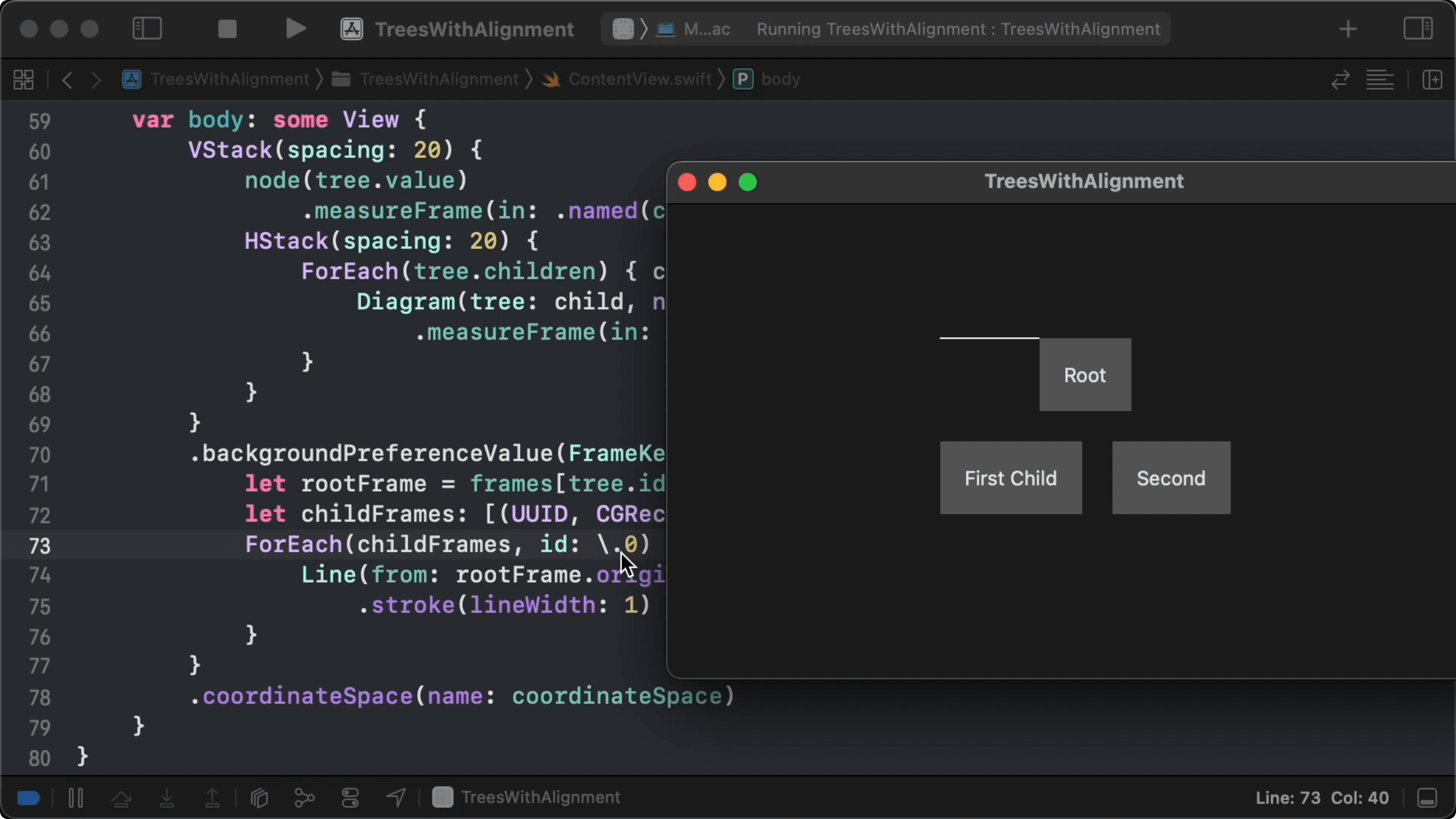15:00 Within the `Diagram` view, we measure the root node's frame, and for each of the children, we recursively create a `Diagram` view and measure its frame. Inside each child's `Diagram` view, there's also a root node measuring its frame and propagating up. By clearing out the `FrameKey` preference value outside the `Diagram` view, we stop the propagation of measured frames from child trees:

``````struct Diagram<A, Node: View>: View {
var tree: Tree<A>
@ViewBuilder var node: (A) -> Node

let coordinateSpace = "diagram"

var body: some View {
VStack(spacing: 20) {
node(tree.value)
.measureFrame(in: .named(coordinateSpace), id: tree.id)
HStack(spacing: 20) {
ForEach(tree.children) { child in
Diagram(tree: child, node: node)
.measureFrame(in: .named(coordinateSpace), id: child.id)
}
}
}
.backgroundPreferenceValue(FrameKey.self) { frames in
let rootFrame = frames[tree.id]!
let childFrames: [(UUID, CGRect)] = frames.filter { \$0.key != tree.id }
ForEach(childFrames, id: \.0) { (_, childFrame) in
Line(from: rootFrame.origin, to: childFrame.origin)
.stroke(lineWidth: 1)
}
}
.coordinateSpace(name: coordinateSpace)
.preference(key: FrameKey.self, value: [:])
}
}
``````

Now the correct lines are drawn: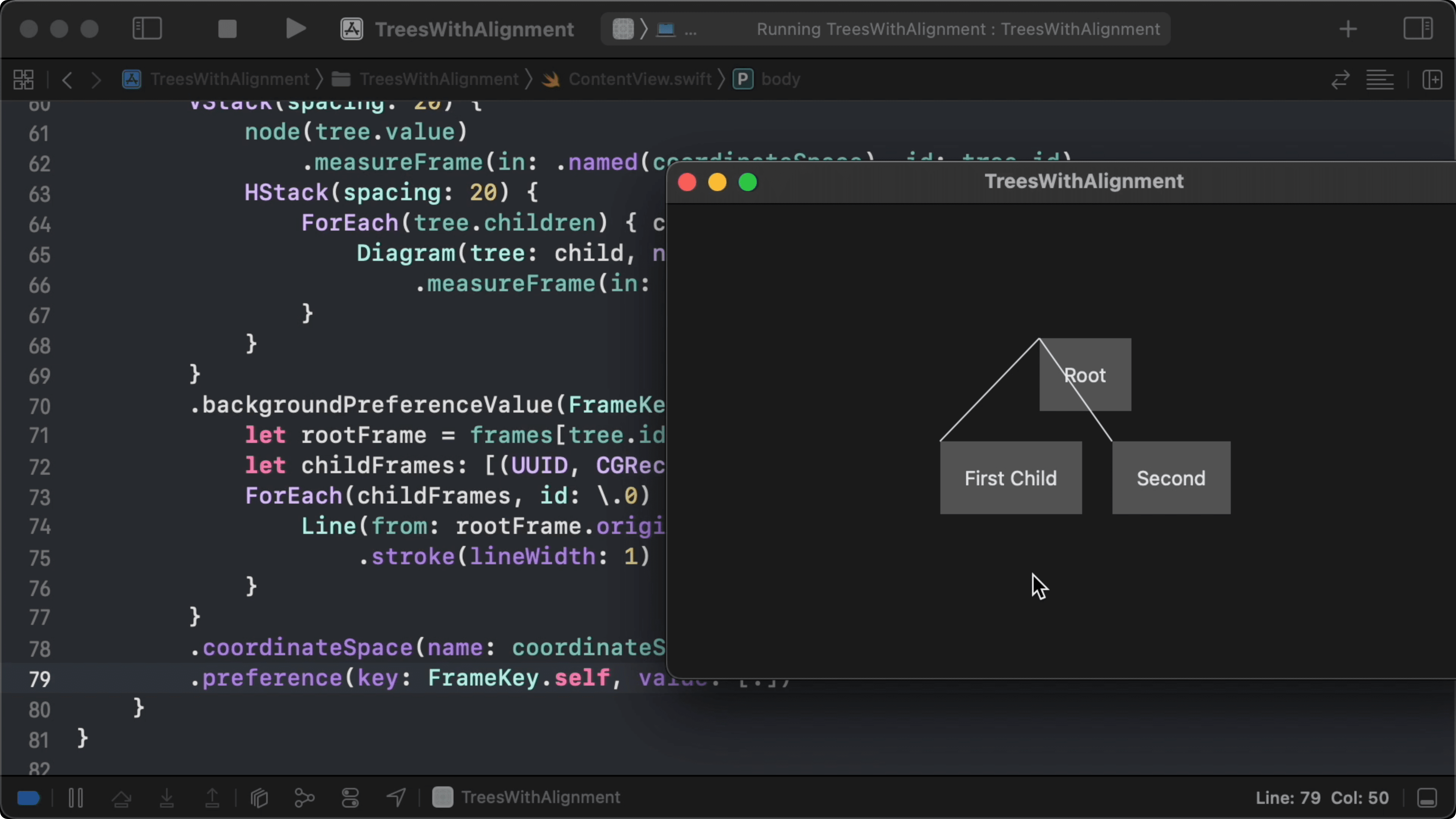16:53 Rather than drawing lines between the frame origins, we want to draw a line from the root node's bottom to the top of each child node. In an extension on `CGRect`, we write a subscript to get the desired point from a frame using a `UnitPoint`, which defines handy static properties such as `bottom` and `top`:

``````struct Diagram<A, Node: View>: View {
// ...
var body: some View {
VStack(spacing: 20) {
// ...
}
.backgroundPreferenceValue(FrameKey.self) { frames in
let rootFrame = frames[tree.id]!
let childFrames: [(UUID, CGRect)] = frames.filter { \$0.key != tree.id }
ForEach(childFrames, id: \.0) { (_, childFrame) in
Line(from: rootFrame[.bottom], to: childFrame[.top])
.stroke(lineWidth: 1)
}
}
.coordinateSpace(name: coordinateSpace)
.preference(key: FrameKey.self, value: [:])
}
}

extension CGRect {
subscript(point: UnitPoint) -> CGPoint {
CGPoint(x: minX + point.x * width, y: minY + point.y * height)
}
}
``````18:07 That doesn't look too bad. But when we give the child nodes strings of different lengths, the tree starts to look a little strange: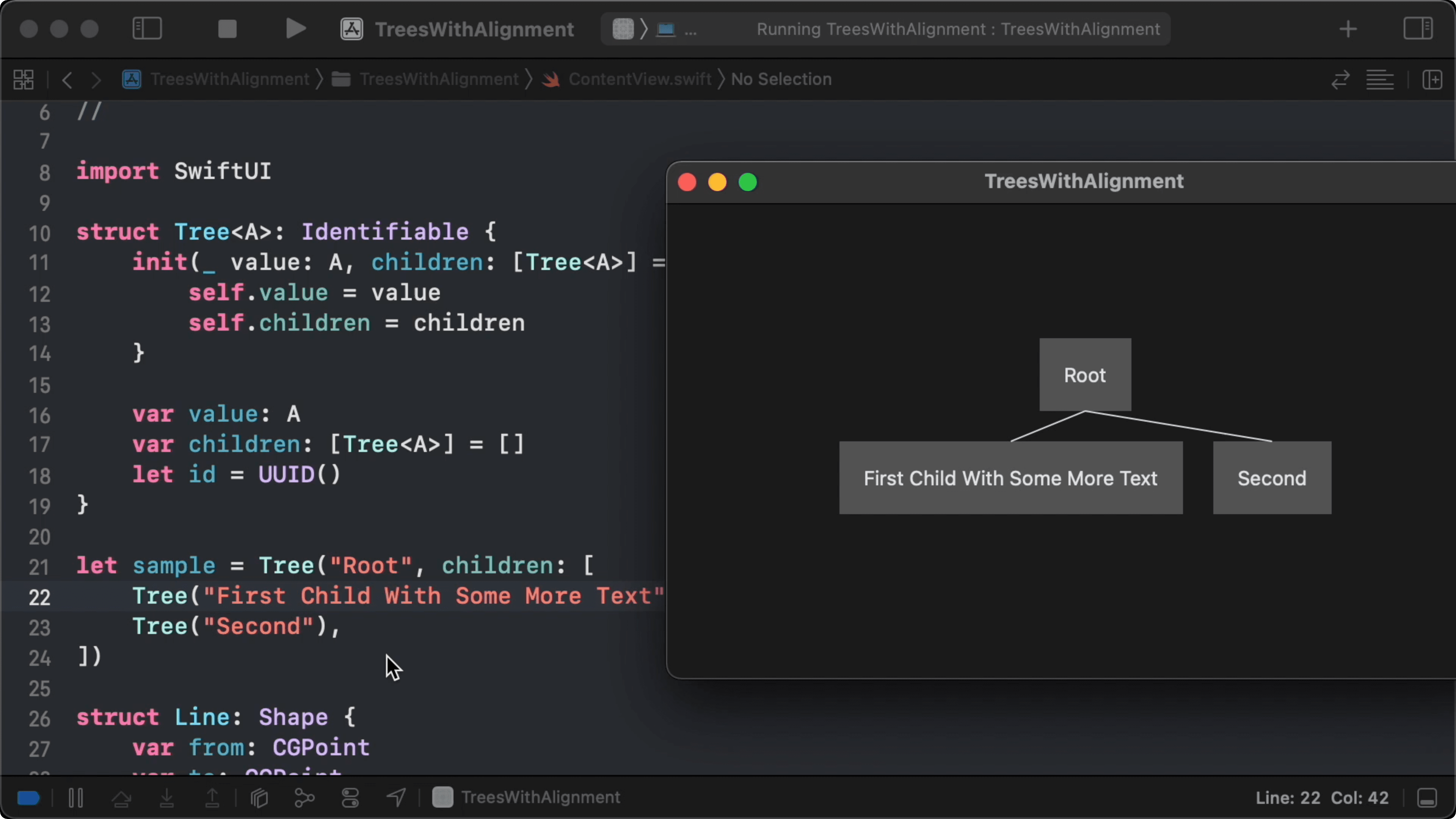18:20 We want the root node to be moved to the right so that the lines to its children become symmetrical. In the next episode, we'll see we can use custom alignment guides to achieve this.

### Resources

• #### Sample Code

Written in Swift 5.5

See All
SwiftUI

### Search for a Mac App: Jumping to Search Results

Episode 317 · Aug 12

SwiftUI

### Search for a Mac App: Generating Search Results

Episode 316 · Aug 05

SwiftUI

### Search for a Mac App: Search Field & Completions

Episode 315 · Jul 29

SwiftUI

### Building a Photo Grid: Refactoring

Episode 314 · Jul 22

SwiftUI

### Building a Photo Grid: Gestures

Episode 313 · Jul 15

SwiftUI

### Building a Photo Grid: Spring Animation (Part 1)

Episode 312 · Jul 08

SwiftUI

### Building a Photo Grid: Gestures

Episode 311 · Jul 01

SwiftUI

### Building a Photo Grid: Animations

Episode 310 · Jun 24

Unlock Full Access

## Subscribe to Swift Talk

• ### Watch All Episodes

A new episode every week